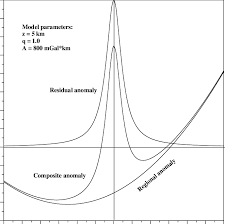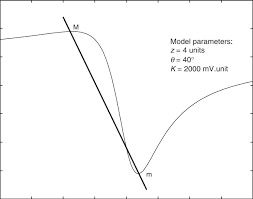## How to Calculate and Solve for Gravity Anomaly for a Semi-Infinite Horizontal Sheet | GravityThe image above represents gravity anomaly for a semi-infinite horizontal sheet.

To compute for gravity anomaly for a semi-infinite horizontal sheet, five essential parameters are needed and these parameters are Gravitational Constant (G), Anomalous Density (Δρ), Thickness of the Sheet (t), Horizontal Distance (x) and Depth Buried (d).

The formula for calculating gravity anomaly for a semi-infinite horizontal sheet:

δgz = 2GΔρt [π/2 + tan-1(x/d)]

Where:

δgz = Gravity Anomaly for a Semi-Infinite Horizontal Sheet
G = Gravitational Constant
Δρ = Anomalous Density
t = Thickness of the Sheet
x = Horizontal Distance
d = Depth Buried

Let’s solve an example;
Find the gravity anomaly for a semi-infinite horizontal sheet when the gravitational constant is 6.67E-11, the anomalous density is 12, the thickness of the sheet is 14, the horizontal distance is 18 and the depth buried is 5.

This implies that;

G = Gravitational Constant = 6.67E-11
Δρ = Anomalous Density = 12
t = Thickness of the Sheet = 14
x = Horizontal Distance = 18
d = Depth Buried = 5

δgz = 2GΔρt [π/2 + tan-1(x/d)]
δgz = 2(6.67e-11)(12)(14) [π/2 + tan-1(18/5)]
δgz = 2.2411200000000002e-8 [1.570 + tan-1(3.6)]
δgz = 2.2411200000000002e-8 [1.570 + 74.475]
δgz = 2.2411200000000002e-8 [76.046]
δgz = 0.000001704

Therefore, the gravity anomaly for a semi-infinite horizontal sheet is 0.000001704 mGal.

## How to Calculate and Solve for Gravity Anomaly for an Infinitely Long Cylinder | GravityThe image above represents gravity anomaly for an infinitely long cylinder.

To compute for gravity anomaly for an infinitely long cylinder, five essential parameters are needed and these parameters are Gravitational Constant (G), Anomalous Density (Δρ), Radius of Cylinder (b), Depth Buried (d) and Horizontal Distance (x).

The formula for calculating gravity anomaly for an infinitely long cylinder:

δgx = GΔρ2πb²d / x² + d²

Where:

δgx = Gravity Anomaly for an Infinitely Long Cylinder
G = Gravitational Constant
Δρ = Anomalous Density
d = Depth Buried
x = Horizontal Distance

Let’s solve an example;
Find the gravity anomaly for an infinitely long cylinder when the gravitational constant is 6.67E-11, the anomalous density is 9, the radius of cylinder is 11, the depth buried is 8 and the horizontal distance is 10.

This implies that;

G = Gravitational Constant = 6.67E-11
Δρ = Anomalous Density = 9
b = Radius of Cylinder = 11
d = Depth Buried = 8
x = Horizontal Distance = 10

δgx = GΔρ2πb²d / x² + d²
δgx = 6.67e-11(9)2π(11)²(8) / (10)² + (8)²
δgx = 6.67e-11(9)2π(121)(8) / (100) + (64)
δgx = 6.67e-11(9)(6082.123) / 164
δgx = 0.000003651098 / 164
δgx = 2.226e-8

Therefore, the gravity anomaly for an infinitely long cylinder is 2.226e-8 mGal.